## 4.3.1 Spliced functions

The definitions of functions can be declared to be valid only within a certain domain of argument space, allowing for error checking when models are evaluated outside their domain of applicability. Furthermore, functions can be given multiple definitions which are specified to be valid in different parts of argument space. We term this function splicing, since multiple algebraic definitions for a function are spliced together at the boundaries between their various domains. The following example would define a function which is only valid within the range:1

truncated_cos(x)[-pi/2:pi/2] = cos(x)


Attempts to evaluate this function outside of the range in which it is defined would return an error that the function is not defined at the requested argument value. Thus, if the above function were to be plotted, no line would be drawn outside of the range. A similar effect could also have been achieved using the select keyword (see Section 3.9.2). Sometimes, however, the desired behaviour is rather that the function should be zero outside of some region of parameter space where it has a finite value. This can be achieved as in the following example:

f(x) = 0
f(x)[-pi/2:pi/2] = cos(x)


Plotting this function would yield the following result: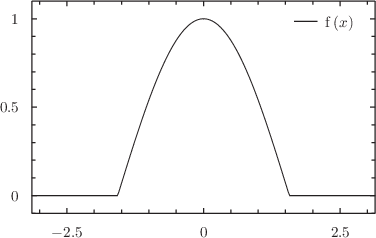To produce this function, we have made use of the fact that if there is an overlap in the domains of validity of multiple definitions of a function, then later declarations are guaranteed take precedence. The definition that the function equals zero is valid everywhere, but is overridden in the regionby the second function definition.

Where functions have been spliced together, the show functions command will show all of the definitions of the spliced function, together with the regions of parameter space in which they are used. This is indicated using the same syntax that is used for defining spliced functions, such that the output can be stored and pasted into a future Pyxplot session to redefine exactly the same spliced function.

When a function takes more than one argument, multiple ranges can be specified, one for each argument. Any of the limits can be left blank if there is no upper or lower limit upon the value of that particular argument. In the following example, the function f(a,b,c) would only be defined when all of a, b and c were in the range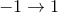:

f(a,b,c)[-1:1][-1:1][-1:1] = a+b+c


Function splicing can be used to define functions which do not have analytic forms, or which are, by definition, discontinuous, such as top-hat functions or Heaviside functions. The following example would define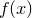to be a Heaviside function:

f(x) = 0
f(x)[0:] = 1


Similar effects may also be achieved using the ternary conditional ?: operator (see Section 7.7), for example:

f(x) = (x>0) ? 1 : 0


Example: Modelling a physics problem using a spliced function

Question
A light bead is free to move from side to side between two walls which are placed atand. It is connected to each wall by a light elastic string of natural length, which applies a force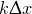when extended by an amount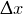, but which applies no force when slack. What is the total horizontal force on the bead as a function of its horizontal position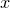?
This system has three distinct regimes. In the region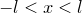, both strings are under tension. When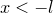, the left-hand string is slack, and only the right-hand string exerts a force. When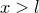, the converse is true: only the left-hand string exerts a force. The case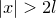is not possible, as the bead would have to penetrate the hard walls. It is left as an exercise for the reader to use Hooke’s Law to derive the following expression, but in summary, the force on the bead can be defined in Pyxplot as:
F(x)[-2*l :-  l] = -k*(x-l)
F(x)[-  l :   l] = -2*k*x
F(x)[   l : 2*l] = -k*(x+l)
where it is necessary to first define a value for l and k. Plotting these functions yields the result: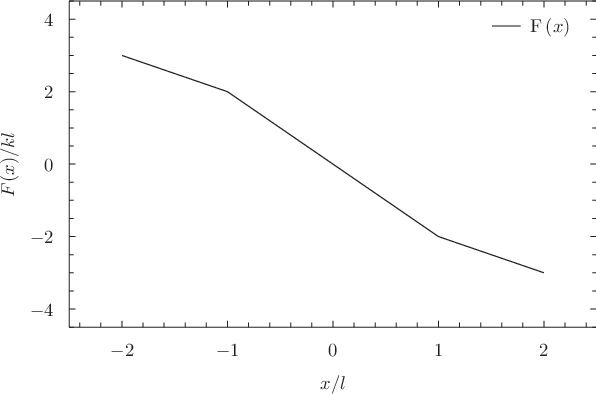Attempting to plot this function with an x-axis which extends outside of the range of values offor which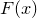is defined, as above, will result in error messages being returned that the function could not be evaluated at all argument values. These can be suppressed by typing (see Section 4.4)
set numeric errors quiet

Example: Using a spliced function to calculate the Fibonacci numbers

The Fibonacci numbers are defined to be the sequence of numbers in which each member is the sum of its two immediate predecessors, and the first three members of the sequence are. Thus, the sequence runs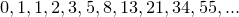. In this example, we use function splicing to calculate the Fibonacci sequence in an iterative and highly inefficient way, hard-coding the first three members of the sequence and then using the knowledge that all of the subsequent members are the sums of their two immediate predecessors:
f(x)     = 0.0
f(x)[1:] = 1.0
f(x)[3:] = f(x-1) + f(x-2)
This method is highly inefficient because each evaluation spawns two further evaluations of the function, and so the number of operations required to evaluate f(x) scales as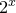. It is inadvisable to evaluate it for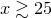unless you’re prepared for a long wait.
A much more efficient method of calculating the Fibonacci numbers is to use Binet’s formula,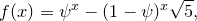where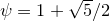is the golden ratio, which provides an analytic expression for the sequence. In the following script, we compare the values returned by these two implementations. We enable complex arithmetic as Binet’s formula returns complex numbers for non-integer values of.
# Binet’s Formula for the Fibonacci numbers
set numerics complex
binet(x) = Re((goldenRatio**x - (1-goldenRatio)**x) / sqrt(5))

set samples 100
set xrange [0:9.5]
set yrange [0:35]
set xlabel "$x$"
set ylabel "$y$"
set key bottom right
plot f(x) , binet(x)Footnotes

1. The syntax [-pi/2:pi/2] can also be written [-pi/2 to pi/2].